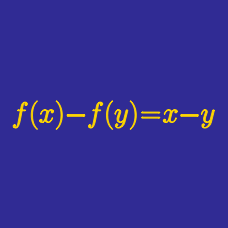Algebra

Functional Equations: Level 3 Challenges

If $f$ is a function satisfying $f(x+y) = 3^yf(x)+2^xf(y)$ for all $x,y \in R$ and $f(1) = 1$, what is the value of $f(3)$?

The polynomial function $f: \mathbb{R}^+ \rightarrow \mathbb{R}^+$ satisfies the functional equation

$f(f(x) ) = 6x + f(x).$

What is the value of $f(17)$?

A function $f: \mathbb{R} \rightarrow \mathbb{R}$ satisfies $f (5-x) = f(5+x)$. If $f(x) = 0$ has $5$ distinct real roots, what is the sum of all of the distinct real roots?

Details and assumptions

The root of a function is a value $x^*$ such that $f(x^*) =0$.

Let a function $f(x)$ has the property:

$f(x+2)= \frac{ f(x)-5}{f(x)-3}.$

Then, which of the following must be equal to $f(2014)$?

Let $f : \mathbb R^+ \rightarrow \mathbb R$ such that $f(x)+2f \left (\frac{1331}{x} \right )=L \cdot x$ where $L$ is a constant.

Knowing that $f(11)=847$, what is $L$?

×